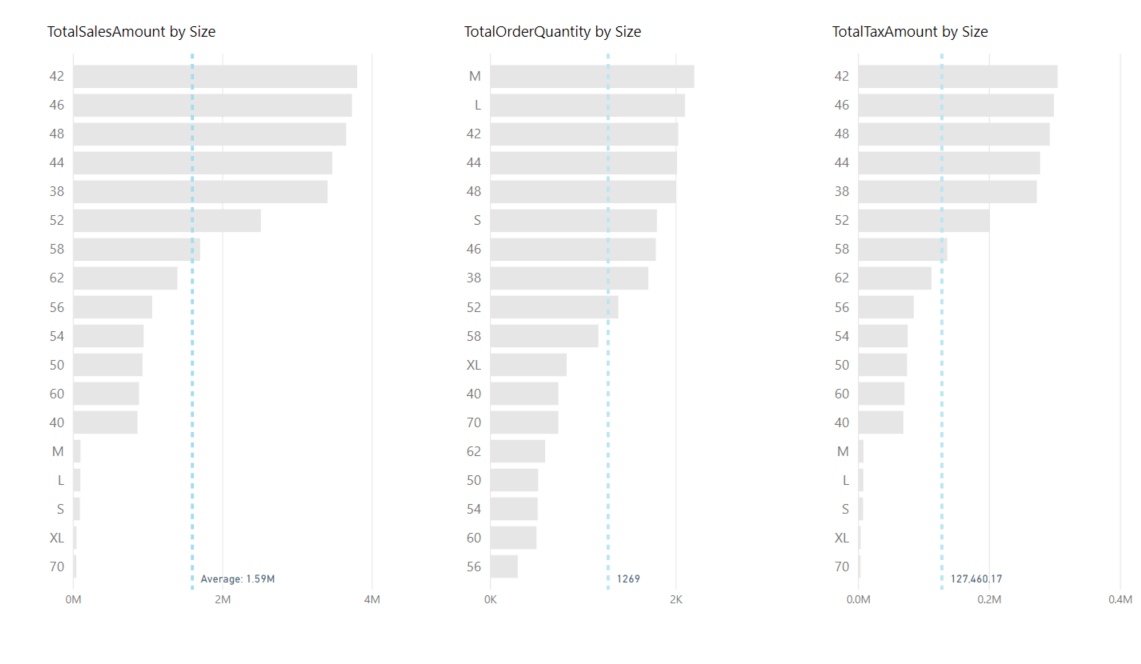#### +61 4 3836 7017

This is the first post in the Strategic colouring in Power BI series. Links to all the posts can be found here

https://www.capstoneanalytics.com.au/master-post-for-strategic-colouring-articles/

So you work in Sales. You want to compare revenue, profit, tax amount over the different business units. You want to see the relative position of a business unit over the other units for the three metrics . How would you do it ? This was precisely what one of our client was after. The following is a solution we provided. The Adventureworks dataset is used in this article to explain the concepts.

We will be visualising SalesAmount, OrderQuantity, and TaxAmt over the Product Size. I have chosen Product Size as the common dimension as there are more than 10 sizes and thus helps to get the point across.

Define 3 measures for the three metrics as follows

TotalSalesAmount =
SUM (  fctSales[SalesAmount] )

TotalOrderQuantity =
SUM ( fctSales[OrderQuantity] )

TotalTaxAmount =
SUM ( fctSales[TaxAmt] )

Next create a new table called ProductSize as follows. The column size will be used as a slicer in our report

ProductSize =
VALUES ( dimProduct[Size] )

Next place a clustered bar chart on a blank page. Drag DimProduct[Size] into the Axis field and drag [TotalSalesAmount] into the Vaue field. Make the data colour “#E6E6E6”. Go to the Analytics pane on the visual and add an Average line and show labels.

Repeat the above for [TotalOrderQuantity] and [TotalTaxAmount]. Your canvas should look like thisThe aim is to apply conditional formatting on the bars so that it gives different colours when a bar is above or below the average, We need to define three measures to calculate the averages.

Average Sales =
CALCULATE (
AVERAGEX (
SUMMARIZE ( fctSales, dimProduct[Size], “SalesTotal”, [TotalSalesAmount] ),
[SalesTotal]
),
ALL ( dimProduct[Size] ),
NOT ( ISBLANK ( dimProduct[Size] ) )
)

Average Orderquantity =
CALCULATE (
AVERAGEX (
SUMMARIZE ( fctSales, dimProduct[Size], “QuantityTotal”, [TotalOrderQuantity] ),
[QuantityTotal]
),
ALL ( dimProduct[Size] ),
NOT ( ISBLANK ( dimProduct[Size] ) )
)

Average Tax =
CALCULATE (
AVERAGEX (
SUMMARIZE ( fctSales, dimProduct[Size], “TaxTotal”, [TotalTaxAmount] ),
[TaxTotal]
),
ALL ( dimProduct[Size] ),
NOT ( ISBLANK ( dimProduct[Size] ) )
)

The NOT ( ISBLANK ( dimProduct[Size] )  is used as I want to remove all the sizes which dont have a value in them

Next we will be defining three measures to get the right colours for the bars

SalesColour =
VAR selsize =
SELECTEDVALUE ( ProductSize[Size] )
RETURN
IF (
SELECTEDVALUE ( dimProduct[Size] ) = selsize
&& [TotalSalesAmount] < [Average Sales],
“#FD625E”,
IF (
SELECTEDVALUE ( dimProduct[Size] ) = selsize
&& [TotalSalesAmount] > [Average Sales],
“#003DFF”,
“#E6E6E6”
)
)

OrderQuantityColour =
VAR selsize =
SELECTEDVALUE ( ProductSize[Size] )
RETURN
IF (
SELECTEDVALUE ( dimProduct[Size] ) = selsize
&& [TotalOrderQuantity] < [Average Orderquantity],
“#FD625E”,
IF (
SELECTEDVALUE ( dimProduct[Size] ) = selsize
&& [TotalOrderQuantity] > [Average Orderquantity],
“#003DFF”,
“#E6E6E6”
)
)

TaxColour =
VAR selsize =
SELECTEDVALUE ( ProductSize[Size] )
RETURN
IF (
SELECTEDVALUE ( dimProduct[Size] ) = selsize
&& [TotalTaxAmount] < [Average Tax],
“#FD625E”,
IF (
SELECTEDVALUE ( dimProduct[Size] ) = selsize
&& [TotalTaxAmount] > [Average Tax],
“#003DFF”,
“#E6E6E6”
)
)

Now we are ready to apply conditional formatting. Select the first chart on the canvas and under Format go to Data colours and click on the three dots under Default colour and click conditional formatting. In the window that comes up select the following options and click OKRepeat the above procedure for the second and third chart and replace SalesColour by OrderColour and TaxColour respectively

The charts are now conditionally formatted. Drag a slicer into the canvas and make it Single select. Drag ProductSize[Size] into the canvas (Remember to drag the right size column, the DimProduct[Size] should not be dragged here).

Select a size in the slicer and see the magic of selective colouring unfold.